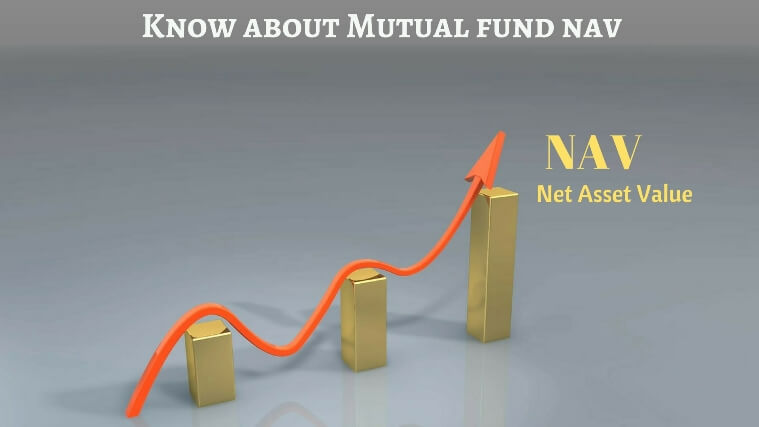BLOG# What you need to know about Mutual Fund NAV?

When you start off with your investing dreams in mutual funds, you tend to come across the term NAV a lot. Quite a few people throw such jargons around their conversation just to make the listener feel that the speaker knows more stuff!
So, what really is NAV? An investment in knowledge pays the best interest- Benjamin Franklin.

It is the acronym for the term ‘Net Asset Value’. It is a number often used in the financial world, especially in mutual fund investments. No need to get scared about this term. Let me explain the term in a simple language. Read ahead.

## So what does NAV mean?

Imagine that you are selling a house. The house is all of 1000 square feet. So, what do you do when you want to sell a house? You enlist the help of a realtor. Let us say, the house fetches 35 lacks Rupees. Once the house is successfully sold, what’s the next step? The real estate agent or the middleman gets a commission. Let us say his cut is Rs.50,000 for his/her services. So, in effect, how much do you get in hand? You get Rs. 34,50,000.

So, one square feet of the house fetches youRs.3,450.

So, the true Value of the house per square feet= (Selling Price – Expenses)/ Size of the house.

Value per square foot= (35,00,000-50,000)/1000 = 3,450.

Similarly, the net asset value per unit of a particular mutual fund scheme is the sum total of the market value of all the assets held in the portfolio INCLUDING cash MINUS expenses DIVIDED by the total number of units held by the investors.

Assume that the market value of the portfolio of a mutual fund scheme is Rs. 100 lakh and the scheme issued 5 lakh units.

The NAV per unit of this particular scheme = (10000000/500000) = Rs. 20.

The market value of a mutual fund scheme may change (and does change) everyday due to various factors and that obviously affects NAV per unit price. Hence, the NAV fluctuates with time. This information is disclosed by the company to the investors, on an everyday basis. This helps investors to know the current status of their holdings in that mutual fund scheme.

## Why is the NAV of a mutual fund scheme important?

1. The NAV number helps the investor in calculating the number of units an investor can buy in a certain scheme.

2. The NAV number helps the investor in calculating redemption amount and profit of an invested amount.

When the investor mentioned in the example above (in point 1) wants to redeem his investment after some time, when the NAV of the said scheme is at Rs. 50(assumption), he/she will receive Rs.50,000 for his/her holding of 1000 units.Hence, the profit per unit, in this investment, is Rs.25

3. The NAV number helps the investor in gauging the general performance of a particular scheme over time. The NAV of a mutual fund scheme is compared at two different time points, to see how well or poorly, the scheme has performed. It is, in effect,an important index of the scheme’s performance.

Assuming the NAV of a scheme is Rs. 25 today and that its NAV2 years back was Rs. 15, the scheme has delivered an absolute return of 66.67 % in two years, from investment to redemption. The annualised growth of this investment is 29.10 %. What does it mean? Each year, this investment has grown by 29.10 %.

We tend to think that the mutual fund scheme with an NAV of Rs.10 is cheaper than the mutual fund scheme with an NAV of Rs. 200. It is not so. Let us see, with a valid example, how and why this is a misconceived notion.

Consider this scenario where you invest Rs. 10,000 each, in scheme A and scheme B. The former fetches 1000 units (10000/10= 1000) and the later fetches 50 units (10000/200= 50). Let us further assume that each of these schemes went up by 20 % in one year. So, each investment has gone up to Rs. 12,000, irrespective of the NAV value. The invested amount and the growth per cent are the factors that determine the returns on investment. The NAV value of the scheme does not affect the growth of the investment.

Returns on a mutual fund investment are higher if the scheme performs better. It is as simple as that.

## Conclusion:

The Net Asset Value (NAV) per unit of a scheme is the price that an investor pays for investing in a particular mutual fund scheme. It is similar to the per square foot rate you get for selling your house, as discussed in the example discussed initially.

So, that must clear the air on the subject of Net Asset Value (NAV) of a mutual fund scheme. If you really want to try a professional advice, now you can enjoy 30 Minutes Complementary Professional Financial Advice by opting for